# Transform Time-Domain Data into Frequency Domain

This example shows how to transform time-domain data into the frequency domain using the FFT block.

Note: To open the example and the associated models, you must have MATLAB® open. Click on the Open Script button while you have this page open on the MATLAB help browser.

Use the Sine Wave block to generate two sinusoids, one at 15 Hz and the other at 40 Hz. Use the Matrix Sum block to add the sinusoids point-by-point to generate the compound sinusoid: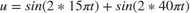Transform this sinusoid into the frequency domain using an FFT block. See the ex_fft_tut model: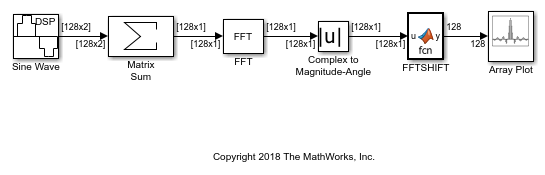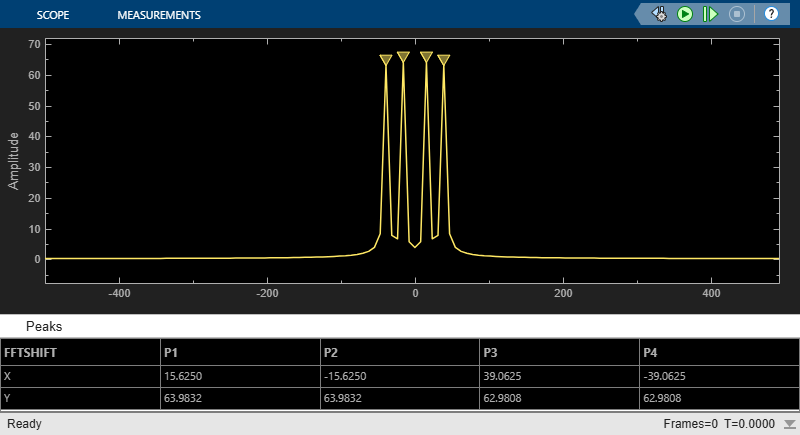The scope shows peaks at 15 and 40 Hz, as expected. You have now transformed two sinusoidal signals from the time domain to the frequency domain.

You can use a Spectrum Analyzer block in place of the sequence of FFT, Complex to Magnitude-Angle, MATLAB Function, and Array Plot blocks. The Spectrum Analyzer computes the magnitude FFT and shifts the FFT internally. See the ex_time_freq_sa model: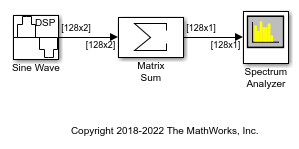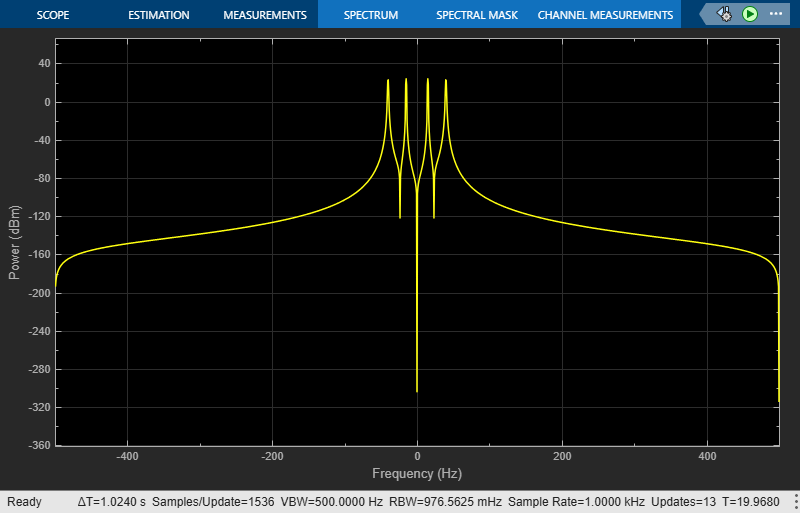The blocks in the Power Spectrum Estimation library compute the FFT internally.